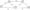# Analysis and Design of Algorithms Jan 2010

Note: Answer any FIVE full questions, selecting at least TWO questions from each part

Part-A

1 (a)Explain theevarious stages of algorithm design and analysis process using a flow chart.

b. Define theef&ilowing:

i)   Spedaiitypes of list.

ii) Paths<and Cycles.

iii)  Setssarrd Dictionaries.

b. Write an algorithm to find the distance between two closest elements in an array of numbers.

2 (a) Prove that :: IE tjCn) e 0(gj(n)) and t2(n) e 0(g2 (n))

then ti(n) + t2(n)€0(max{g1(n),g2(n)})

(b) Write an algorithm to compute n! recursively. Set up a recurrence relation for the algorithm’ssbasic operation count and solve it.

(c)Explain the^ method of comparing the order of the growth of 2 functions using limits. Compare order of growth of log2 n and Vn.

3 a. Discuss how/quick sort works to sort an array and trace for the following data set. Draw the tree of recursive calls made* 1, 1, 9, 9, 5, 5, 6, 6

b. What is stabie. algorithm? Is Quick Sort stable?

c.   Write the algorithm for binary search and find the average case efficiency.

4 a. Explain the.^difference between DFS and BFS. Solve topological sorting problem using DFS algorithm, with an example.

b. Show the steps in multiplying the following 2 integers using efficiency integer multiplicatiommethod: 5673 x 6342.

Part — B

5 a. What is an AML tree? Explain the need for rotation of AVL tree. Construct an AVL tree for the list 105 2Q]30, 25,27, 7,4 by successive insertion.

b. Write an algorithm for comparison counting and show how comparison counting method sorts the list£;45, 2,19,10, 33, 22,1,23

6  a. Explain hashing and various collision resolution techniques.

b. Solve the alli^airs shortest path problem for the diagraph with the weight matrix.

c. Using dynamic:programming, solve the following knapsack instance: n = 3,[©1,co.2:?jca3]= [l,2,2] and [PpP2,P3] = [18, 16, 6] and M = 4

7 a. Solve the following instances of the single source shortest path problem with vertex ‘a’ as the source.Fig.Q7(a)

b- Write the Kruskal’s algorithm to find the minimum cost spanning tree. Also trace the algorithm for the graph of figure Q7 (b).

c.   What are Huffman codes and trees? Discuss the advantage of Huffman’s code.

8  a. Discuss p and np problems.

b. What is the central principle of back tracking? Taking n-queens problem as an example, explain the solution process.

c.   What is branch and bound? How is it different from back tracking?

d.  Draw the state space tree for the sum of subset problem of the instance: